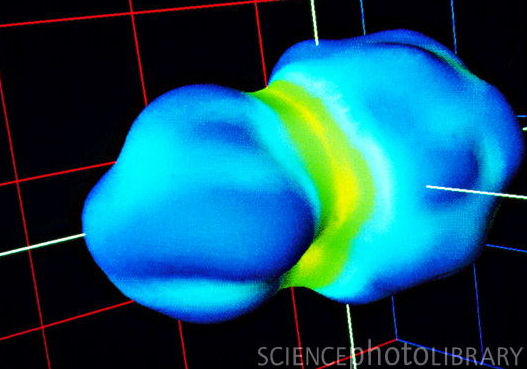Uranium nuclei

# Are These Calculations Correct?

 p { font: 16pt "verdana"} Here's something: If you have a concept of the size of an atom, and know that the atomic nucleus is about 10,000 times smaller  with a volume a one-trillionth that of an atom  then you should appreciate how much force it would take to push two uranium nuclei right up against each other. It's slightly less than 2,000 lbs! I happened to calculate this because the question came to mind while I was watching a show about the Large Hadron Collider. I had estimated a millionth of a pound, maybe even on the order of a thousandth of a pound, but, boy, was I surprised. I'm still not convinced my calculation is correct, even after posting it on Physics Forum and asking several physics-trained people about it and getting no objections. Here are some specifics on how I did the calculation: I Googled up "nuclear diameter" and got 15 femtometers (15x10-15 meter) from Wikipedia. Here's a diagram of two uranium nuclei pushed right up against each other:And here, below, from Google Images, is someone else's view of two nuclei, doing something, approaching or receding:Or maybe they're fissioning . . . That ~2,000 lbs of repelling force between two touching uranium nuclei, I used Coulomb's Law, which is simple enough, and I assumed 92 positively charged protons in each nucleus, each having an electric charge of 1.902x10-19 coulomb, for a total charge, each nucleus, of 1.47x10-17 coulomb  with the distance between the charge centers being 15x10-15 meter. See below, Uranium Nuclei Calculation. THEN I got inspired to do a second unbelievable calculation, namely the attracting force that would act between all the electrical charges in a copper penny if the electrons could be separated from the protons and arranged in two penny-sized disks. The mass of a copper penny (3.1 grams) is from hypertextbook.com, and the thickness (1.55 mm) is from Wikipedia. The force of attraction comes to slightly more than the weight of the earth! Here's a diagram of how I was imagining the protons and electrons being arranged:THE VARIABLES: The atomic weight of copper is 63.546, therefore  from Avogadro and all that  the number of copper atoms = 2.94x1022. Each copper atom has 29 protons and 29 electrons which, times 8.52x1023 protons and the same number of electrons, each having a charge of 1.602x10-19, gives a total charge, positive and negative, of: 1.602x10-19 x 8.52x1023 = 136,436 coul.
 That's a stupendous amount of charge. So, how much force is needed to hold that much positive charge 1.55 millimeters from that much negative charge? (If you could put that many coulombs of charge in a capacitor the size of a penny, man, THAT would be useful!) Using Coulomb's Law, the force of attraction between the disk of electrons and the disk of protons comes to 6.97x1025 Newtons. If you divide that by the gravitational constant, 9.8 m/s2, you get 7.12x1024 kg. That's slightly more than the mass of the earth (6×1024 kgs)! NOTE THIS: The force of 6.97x1025 Newtons x 1.55 mm = 1.08x1023 Joules. That's the potential energy between the disk of electrons and the disk of protons. That many Joules is the amount of energy used by all humanity at its current rate (13 trillion watts) in 264 years. At the price Pepco charges for energy (~15 cents per kWh), it's worth \$4.5 quadrillion. It's tempting to think that that much energy is in a penny. But  and assuming my calculations are correct  it actually represents how much effort would be needed to separate all the electrons in a copper penny 1.55 mm from all the protons. Uranium Nuclei Calculation: The calculation for the two uranium nuclei goes like this: The electrical charge of each nucleus is 92 times the charge of each proton, or 92(1.602x10-19 coulomb) = 1.47x10-17 coulomb The force between electrically charged objects is described by Coulomb's Law, namely, Force = k(q1 x q2)/d2
 where,
 q1 = q2 = 1.47x10-17 coulomb k = Coulomb's Constant = 9x109 N-m2/coulomb 2 d = 15x10-15 meter
 Just plug and chug. But I'm still wondering: Are electrical forces REALLY THIS POWERFUL?     Bob Global warming is the increase in the average temperature of Earth's near-surface air and oceans since the mid-20th century and its projected continuation  Wikipedia.